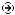YOU CAN CODE!With The Case Of UCanCode.net  Release The Power OF  Visual C++ ! Home |  Products | Purchase |  Support | DownloadsDownload EvaluationPricing & Purchase?E-XD++Visual C++/ MFC ProductsOverviewElectronic Form SolutionVisualization & HMI SolutionPower system HMI SolutionBar code labeling SolutionWorkflow SolutionCoal industry HMI SolutionInstrumentation Gauge SolutionReport Printing SolutionGraphical modeling SolutionGIS mapping solutionVisio graphics solutionIndustrial monitoring SolutionFlowchart and diagramming SolutionOrganization Diagram SolutionGraphic editor Source CodeUML drawing editor Source CodeMap Diagramming SolutionArchitectural Graphic Drawing SolutionRequest EvaluationActiveX COM ProductsOverviewTechnical Support# Get Ready to Unleash the Power of UCanCode .NET

UCanCode Software focuses on general application software development. We provide complete solution for developers. No matter you want to develop a simple database workflow application, or an large flow/diagram based system, our product will provide a complete solution for you. Our product had been used by hundreds of top companies around the world!

"100% source code provided! Free you from not daring to use components because of unable to master the key technology of components!"

CAM simulator Sample with VC++ MFC Source Code## Introduction

After 6 months of work, here is finally my first MFC program release. This program is a CAM simulator. I am not a software engineer, so please forgive me for errors. If you have questions please let me know. I am sure that you will find them. The lecture of data files was a big problem to me at the beginning, so here is the implementation. I needed to read *.txt files, what the CNC machine generate. Here is an example M X25 Y23 Z25 SPEED1500. This is a block of data, needed to setup the machines. This is a kind of language that the machine use to move on the coordinates over the work space. So in this implementation you can read a full document, splitting the block in the necessary X Y Z and SPEED values. This is my first upload, I have more things to say about this program I will try to improve soon, this short commentary.Collapse
```void CProgramaDoc::OnFileOpen()
{
CString fichero="archivo de Código G",temp;

CFileDialog archivos(true,NULL,fichero,OFN_ALLOWMULTISELECT,
"Codigo G (*.tap)|*.tap|Codigo G (*.act)|*.act||",NULL);

if (archivos.DoModal() == FALSE) return;
fichero=archivos.GetPathName();

CFile cfFile (fichero, CFile::modeNoTruncate | CFile::modeRead);

nl=0;
do
{
if(temp.GetLength() == 0) continue;
strcpy(linea[nl],temp);
nl++;

int i,j,k,d,sp;
char aux;

for ( i=0; i<5000; i++)
{
x[i] = y[i] = z[i] = 0;
}

kn=0;
for (i=0;i<nl;i++)
{

if (linea[i]=='S');
{
Inicio=1;
}

if (linea[i]=='M');
{
sp = 0;
k = 0;
d = strlen(linea[i]);

for (j=0;j<d;j++)
{
if ((linea[i][j]=='-')|(isdigit(linea[i][j]))|
(linea[i][j]=='.'))
{
aux[k] = linea[i][j];
k++;
continue;
}

if (linea[i][j]==' ')
{
sp++;
if (sp==1) continue;

aux[k]=0;

if (sp==2)
if ((aux=='-')|(isdigit(aux))|(aux=='.'))
x[kn] = atof(aux);
else
x[kn] = x[kn-1];

if (sp==3)
if ((aux=='-')|(isdigit(aux))|(aux=='.'))
y[kn] = atof(aux);
else
y[kn] = y[kn-1];

if (sp==4)
if ((aux=='-')|(isdigit(aux))|(aux=='.'))
z[kn] = atof(aux);
else
z[kn] = z[kn-1];

k = 0;
continue;
}
}

kn++;
j = d;
}
}

presentar = 1;

//    VERIFICANDO TORNO O FRESA

sumz = 0;
for (i=0; i<nl; i++)
{
sumz += abs(z[i]);
}

if (sumz == 0)
torno = 1;
else
fresa = 1;

UpdateAllViews(NULL);

}
```